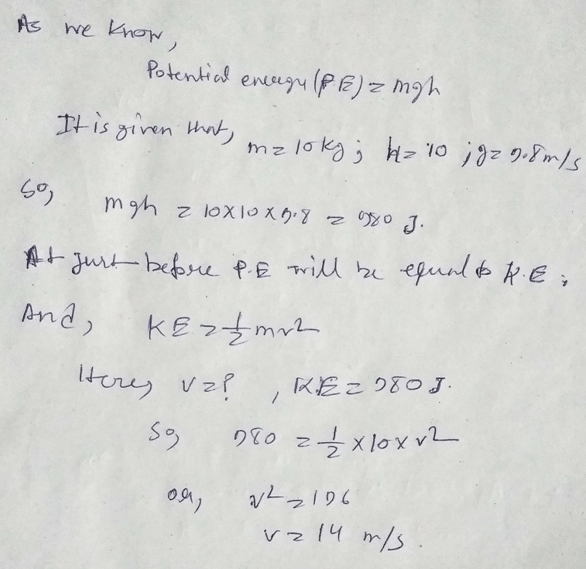# Telangana SCERT Class 9 Physical Science Chapter 10 Work And Energy Solution

Telangana SCERT Class 9 Physical Science Chapter 10 Solution – Work And Energy. Here in this post we provides Class 9 Physical Science Work And Energy Telangana State Board Solution. Telangana State Board English Class IX Medium Students can download this Solution to Solve out Improve Your Learning Questions and Answers.

## Telangana State Board Class 9 Physical Science Chapter 10 Work And Energy Solution:

### Work And Energy

1) What is work according to science and write its units.

Ans: – The work is denoted by the displacement of moving some weight from one placement to others places. It unites is joule.

2) state few examples where displacement of an object is in the direction opposite to the force acting on the

Ans: – There are various example of opposite displacement of an objet like if we through a ball into the sky, the object is going speedily against the friction forces on a floor.

3) Write few daily life examples in which you observe conservation of energy.

Ans: – There are various example of conservation of energy in our daily life like if we hit a ball then it roles out here the forces converted to kinetic energy as we all know that energy can’t be destroyed or created it just changes from one form to another.

4) Give some examples for renewable sources of energy.

Ans: – The examples of renewable energy are sunlight, tidal energy, hydroelectric power plant, wind energy etc.

Application of concepts

1)A man carrying a bag of total mass 25kg climbs up to a height of 10m in 50 seconds. Calculate the power delivered by him on the bag.

Ans: – As kinetic energy= m×g×h,

Here m= mass= 25kg, g=9.8, h=10m.

So, energy=25 × 9.8 × 10= 2450.

And, power= energy/time= 2450/50=49j/s.

2) A 10 kg ball is dropped from a height of 10m. Find (a) the initial potential energy of the ball, (b) the kinetic energy just before it reaches the ground, and (c) the speed just before it reaches the ground.

Ans: –3)Calculate the work done by a person in lifting a load of 20 kg from the ground and placing it 1m high on a table.

Ans: – As potential energy= mass× gravitation × height.

So, potential energy= (20×9.8×1)= 196J.

4)Find the mass of a body which has 5J of kinetic energy while moving at a speed of 2m/s.

Ans: – KE = 1/2mv^2;

Here, KE=5J, m=mass=?, v=2m/s.

So, 5=1/2m×4,

Or, m=2.5kg.

5) A cycle together with its rider weighs 100kg. How much work is needed to set it moving at 3 m/s.

Ans: – As KE=1/2mv^2;

Here, KE=?, m=mass=100kg, velocity=3m/s.

So, kinetic energy= ½×100×9;

Or, KE=450J.

6) Which of the renewable sources of energy would you think suitable to produced in you native place? Why?

Ans: – Do yourself.

Higher Order Thinking Questions

1)When you lift a box from the floor and put it on an almirah the potential energy ofthe box increases but there is no change in its kinetic energy. Is it violation ofconservation of energy? Explain.

Ans: – As we all know that the sum of potential and kinetic energy always be same according to conservation of energy. For the lifting of the box to the almirah the equal and opposite forces are working in this activity so the velocity remains constant and if there are no changes in velocity energy will be constant.

2)When an apple falls from a tree what happens to its gravitational potential energy just as it reaches the ground? After it strikes the ground?

Ans: – As we all know energy doesn’t created or destroyed its just changes from one form to another so this gravitational energy will be converted to the kinetic energy when it falling from tree and after touching the ground it will converted to heat energy.

MULTIPLE CHOICE QUESTIONS.

1.) S.I. unit of work

a) N-m b) Kg-m c) N/m d) N-m2.

Ans: – option (a).

2.) The energy possessed by a body by virtue of its motion is called as

a) Potential energy b) Kinetic energy

c) Attractive energy d) Gravitational energy.

Ans: – option (b).

3.) A person is climbing a ladder with a suitcase on his head. Then the work done by thatperson on that suitcase is

a) Positive b) Negative c) Zero d) Can not be defined.

Ans: – Option (c).

4.) If you have lifted a suitcase and kept it on a table., then the work done by you will depend on

a) The path of the motion of the suitcase

b) The time taken by you to do the work

c) Weight of the suitcase